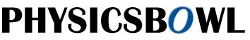### Exam Conventions

• Is there an equation sheet?
YES!  The equation sheet is provided to students.  However, it does NOT include all possible formulas that might be useful on the contest... it has many of the basic equations but it is not, nor is it intended to be, an exhaustive list of what may be used on the questions.

The equation sheet has changed over time with many changes coming in both 2008 and 2009.  The following is a link to the most recent version of the equation sheet. Equation Sheet for 2023

The next link is an "annotated" equation sheet explaining what each of the equation represents... this is NOT the equation sheet provided on the contest. Annotated Equation Sheet
• What of constants?
As it is with the equation sheet, some constants are provided.  All constants on the sheet are used in the construction of the exam.  For all questions related to gravity, use g = 10 m/s2.

Again, this is NOT an exhaustive list of all constants, but the ones most commonly used.  Values such as the resistivity of copper would not be expected of students... but perhaps something qualitative about such a value would be expected (i.e., is the resistivity greater for copper or glass)?

The constants sheet has undergone changes in the past couple of years. The following is a link to the most recent version of the constants sheet.
Constants Sheet for 2023
• What assumed conventions are used on the exam?
The assumptions used in the construction of the contest are those typically used in testbooks.  For clarity, we try to make implicit assumptions clear in the stem of the question...

Here are some of the working assumptions:
• g = 10 m/s2

• All currents are conventional unless otherwise noted.

• "A person launches an object from the top of a 10 meter high building..." assumes that the object is 10 meters off the ground when thrown... if the height of the person is required for the question, it will be made clear from the context.

• While important in science, unless otherwise noted, significant figures are not considered in the problem (although we try to keep things reasonable).

• All masses are rest masses (unless otherwise noted).